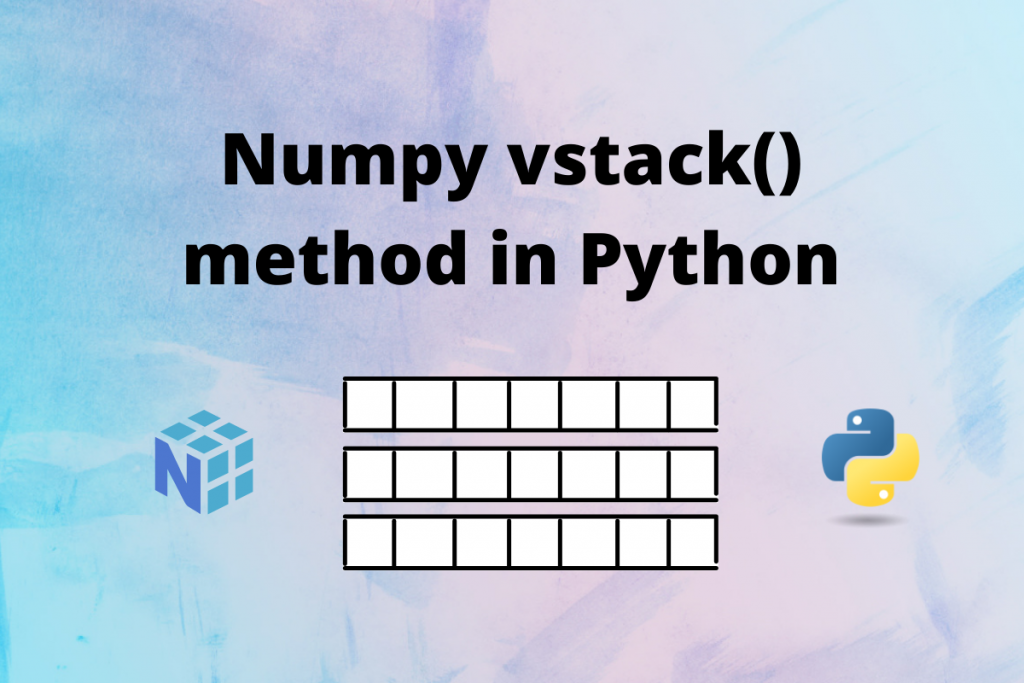# Numpy vstack() method – A Complete OverviewHello everyone! In this tutorial, we will learn what the Numpy `vstack()` method is and how to use it in Python. So let’s get started.

## What is numpy.vstack() method ?

`Numpy.vstack()` is a function in Python that takes a tuple of arrays and concatenates them vertically along the first dimension to make them a single array.

It’s syntax is:

```numpy.vstack(tup)
```

The parameter it takes is a tuple which is a sequence of ndarrays that we want to concatenate. The arrays must have the same shape along all axis except the first axis.

The method returns a ndarray which is formed by stacking the arrays given in the input. The returned array will have at least 2 dimensions.

## Examples of Numpy vstack()

For linear 1-D arrays, all the arrays are stacked vertically to form a 2-D array. All the input arrays must have the same length.

```import numpy

a = numpy.array([1, 2, 3, 4, 5])
b = numpy.array([6, 7, 8, 9, 10])
c = numpy.array([11, 12, 13, 14, 15])

print("Shape of array A:", a.shape)
print("Shape of array B:", b.shape)
print("Shape of array C:", c.shape)
print()

stack = numpy.vstack((a, b, c))
print("Shape of new stacked array:", stack.shape)
print("Stacked array is")
print(stack)
```
```Shape of array A: (5,)
Shape of array B: (5,)
Shape of array C: (5,)

Shape of new stacked array: (3, 5)
Stacked array is
[[ 1  2  3  4  5]
[ 6  7  8  9 10]
[11 12 13 14 15]]
```

For N-dimensional arrays, arrays are stacked along the first dimensions as shown in the following example.

```import numpy

a = numpy.array([ [1, 2, 3], [4, 5, 6] ])
b = numpy.array([ [7, 8, 9], [10, 11, 12] ])

print("Shape of array A:", a.shape)
print("Shape of array B:", b.shape)
print()

stack = numpy.vstack((a, b))
print("Shape of new stacked array:", stack.shape)
print("Array is")
print(stack)
```

Output:

```Shape of array A: (2, 3)
Shape of array B: (2, 3)

Shape of new stacked array: (4, 3)
Array is
[[ 1  2  3]
[ 4  5  6]
[ 7  8  9]
[10 11 12]]
```

For N-dimensional arrays, the shape of the arrays must be the same along all dimensions except the first dimension as shown below.

```import numpy

a = numpy.array([ [1, 2], [3, 4] ])
b = numpy.array([ [5, 6], [7, 8], [9, 10] ])

print("Shape of array A:", a.shape)
print("Shape of array B:", b.shape)
print()

stack = numpy.vstack((a, b))
print("Shape of new stacked array:", stack.shape)
print("Array is")
print(stack)
```
```Shape of array A: (2, 2)
Shape of array B: (3, 2)

Shape of new stacked array: (5, 2)
Array is
[[ 1  2]
[ 3  4]
[ 5  6]
[ 7  8]
[ 9 10]]
```

## Conclusion

In this Python tutorial, we learned about `vstack()` method present in the NumPy module. This function makes most sense for arrays with up to 3 dimensions. For instance, for pixel-data with a height (first axis), width (second axis), and r/g/b channels (third axis).